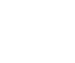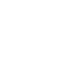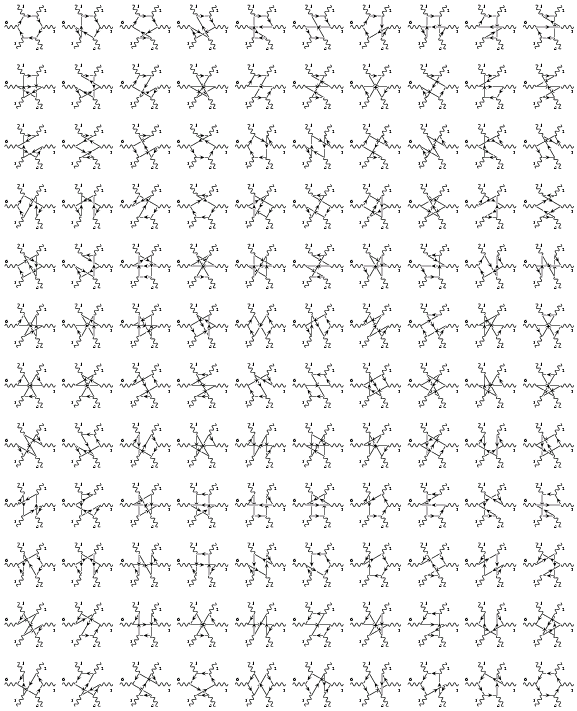Example: 6-Photon Scattering This example, by Greg Mahlon, shows that 120 diagrams can be generated with a relatively small program. Try doing that with a GUI drawing program!```/* Draw all 120 six-photon scattering diagrams on a single * page in (nearly) unreadable form * * by Greg Mahlon */ #include /* some parameters */ #define RAD 2.8 #define PHLEN 3.2 #define ARROWANGLE 15 #define DELTA 1.25 #define XMIN 0.0 #define YMAX 10.08333 #define XWIDTH 0.80 #define YWIDTH 0.83 main() { page pg; FeynDiagram *FD; line_plain *L; line_wiggle *PHOTON; xy vtx; text *LABEL; int row=0; int column=0; int fdnum=0; int i,p1,p2,p3,p4,p5; int j1,j2; int perm; perm = 0; perm = 0; for (p1=1; p1<6; ++p1) { for (p2=1; p2<6; ++p2) { if (p2 != p1) { for (p3=1; p3<6; ++p3) { if ( p3 != p1 && p3 != p2 ) { for (p4=1; p4<6; ++p4) { if ( p4 != p1 && p4 != p2 && p4 != p3 ) { for (p5=1; p5<6; ++p5) { if ( p5 != p1 && p5 != p2 && p5 != p3 && p5 !=p4) { /* we have found a new permutation of 12345 */ perm = p1; perm = p2; perm = p3; perm = p4; perm = p5; fdnum = 10*row + column; FD[fdnum] = new FeynDiagram(pg, XMIN+column*XWIDTH, XMIN+DELTA+column*XWIDTH, YMAX-row*YWIDTH); FD[fdnum]->line_wiggle_width.scale(.75); FD[fdnum]->fontsize.set(1.10); /* vertex locations */ for (i=0; i<6; ++i) { vtx[i][fdnum] = polar(RAD, 120-60*i); } /* fermion loop (changes with diagram) */ for (i=0; i<6; ++i) { j1 = perm[i]; j2 = perm[i+1]; L[i][fdnum] = new line_plain(*FD[fdnum], polar(RAD,120-60*j1), polar(RAD,120-60*j2)); } /* photons (stay the same) */ for (i=0; i<6; ++i) { PHOTON[i][fdnum] = new line_wiggle(*FD[fdnum], polar(RAD,120-60*i), polar(RAD,120-60*i) +polar(RAD,120-60*i)); } /* photon labels (stay the same) */ LABEL[fdnum] = new text(*FD[fdnum], "1",xy(-1.8,5.0), 0.,1.,0.); LABEL[fdnum] = new text(*FD[fdnum], "2",xy(3.0,4.0), 0.,1.,0.); LABEL[fdnum] = new text(*FD[fdnum], "3",xy(5.0,-0.7), 0.,1.,0.); LABEL[fdnum] = new text(*FD[fdnum], "4",xy(1.8,-5.0), 1.,0.,0.); LABEL[fdnum] = new text(*FD[fdnum], "5",xy(-3.0,-4.0), 1.,0.,0.); LABEL[fdnum] = new text(*FD[fdnum], "6",xy(-5.0,0.7), 1.,0.,0.); /* diagram is DONE -- increment position */ ++column; if ( column == 10 ) { ++row; column = 0; } } } } } } } } } } pg.output(); return 0; } ```